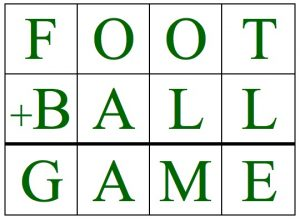Replace the letters in FOOT + BALL = GAME with numbers to make the sum correct. Each letter represents a different digit.

Here is one example: F=1, O=9, T=4, B=6, A=5, L=3, G=8, M=2, E=7

How many solutions can you find?

There are 224 solutions altogether.

You can work with pencil and paper or you might want to use scrap card and make 20 cards, 2 for each digit, all the same size. Then you can try to find solutions by re-arranging 12 of the cards in a 3 by 4 array. Work systematically so you don’t keep trying the same arrangement.

Why not do this as a family group or class investigation? Put a chart on the wall and write up the new solutions as learners find them?

 Examples: F=2, O=9, T=7, B=3, A=4, L=1, G=6, M=0, E=8 2997 3411 + 6408 Replace B,A,L,L here: 2004 BALL + 5981 Replace T, L and E here: 199T 653L + 852E Now find some solutions of your own.
Tagged with: